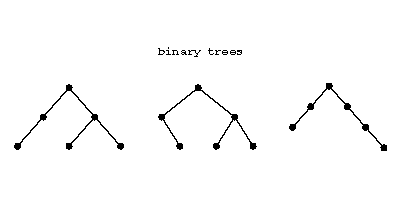Binary tree

A (planar) rooted tree for which every node has a left child, a right child, neither, or both. Three examples are:Figure: b110530a

These three are all different.

The number of binary trees with $n$ nodes, $p$ left children, $q$ right children ($p+q=n-1$) is

$$\frac1n\binom np\binom{n}{p+1}=\frac1n\binom np\binom nq.$$

The numbers $n^{-1}\binom np\binom{n}{p+1}$ are called Runyon numbers or Narayama numbers.

A complete binary tree is one in which every node has both left and right children or is a leaf (i.e., has no children). E.g., there are two complete binary trees with five nodes:Figure: b110530b

A complete binary tree has an odd number of nodes, say $2k+1$, and then the number of leaves is $k+1$. Label the $k+1$ leaves from left to right with symbols $x_1,\ldots,x_{k+1}$. Then the various complete binary trees with their $k+1$ leaves labelled in this order precisely correspond to all the different ways of putting brackets in the word $x_1\ldots x_{k+1}$, each way corresponding to a computation of the product by successive multiplications of precisely two factors each time. The number of ways of doing this, and hence the number of binary trees with $k+1$ nodes, is the Catalan number

$$\frac{1}{k+1}\binom{2k}{k},k=1,2,\ldots.$$

The problem of all such bracketings of a product (of numbers) was considered by E. Catalan in 1838 [a1].

The correspondence between complete binary trees and (complete) bracketings gives a bijection between complete binary trees with leaves labelled with elements from a set $X$ and the free magma on $X$.